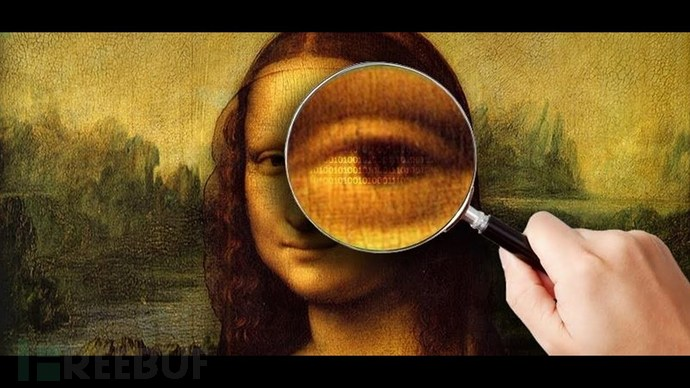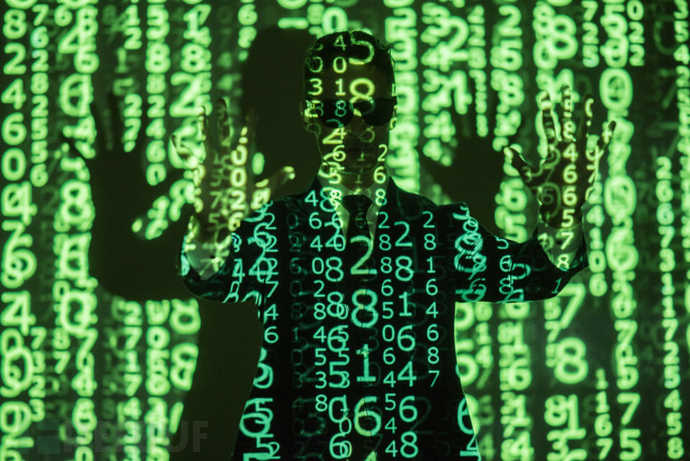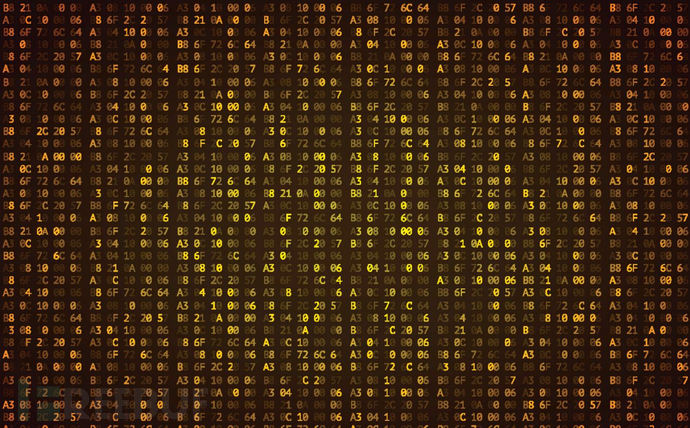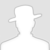FreeBuf.COM网络安全行业门户，每日发布专业的安全资讯、技术剖析。FreeBuf+小程序

Steganographer：一款能够帮助你在图片中隐藏文件或数据的Python隐写工具
2020-07-26 18:44:40## Steganographer

Steganographer是一款功能强大的隐写工具，该工具基于Python编程语言开发，能够帮助广大研究人员在一张图片中实现数据或文件的隐写。这个Python模块可以将文件隐藏在一张图片之中（当前版本仅支持PNG文件），并将包含了隐写数据的文件导出至磁盘中存储。可隐写的最大文件大小取决于图片的尺寸。计算方式如下：

`max_file_size = ( height_of_image * width_of_image * 6 / 8 ) bytes`

## 工具下载&使用

`git clone https://github.com/priyansh-anand/steganographer.git`

## 工作机制`a_pixel = (17,32,11)     # (RED, GREEN, BLUE)`

`max_file_size = ( height_of_image * width_of_image * 6 / 8 ) bytes`

`binary_data = 0b100111`

```a_pixel = (0b10001, 0b100000, 0b1011)   # binary representation of a_pixel values

# Let's change a_pixel's RED Channel

# 0b10001 -> 0b10010  ( First 2 bits are 10 )

a_pixel = (0b10010, 0b100000, 0b1011)  # modified pixel```

```a_pixel = (0b10010, 0b100000, 0b1011)

# Let's change a_pixel's GREEN Channel

# 0b100000 -> 0b100001  ( The 2 bits are 01 )

a_pixel = (0b10010, 0b100001, 0b1011)  # modified pixel```

```a_pixel = (0b10010, 0b100001, 0b1011)

# Let's change a_pixel's BLUE Channel

# 0b1011 -> 0b1011  ( The 2 bits are 11 ) ; Notice that the value wasn't changed this time

a_pixel = (0b10010, 0b100001, 0b1011)  # pixel wasn't modified this time```

```a_pixel             = (0b10001, 0b100000, 0b1011) = (17, 32, 11)

a_pixel_with_data   = (0b10010, 0b100001, 0b1011) = (18, 33, 11)```## 许可证协议

Steganographer项目的开发与发布遵循MIT开源许可证协议。

## 项目地址

Steganographer：【GitHub传送门

# 图像处理 # 隐写 # 图像算法

+ 收入我的专辑• 0 文章数
• 0 评论数
• 0 关注者